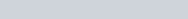# Reflected motion

This post was inspired by Chandru Iyer’s post here.

Consider a light ray sent a certain distance s that is immediately reflected back. According to Newtonian mechanics if a light ray travels at speed c, then for a body moving at speed v relative to the stationary frame, the light ray should travel at the speed c − v one way and at speed c + v the other way.

The total distance of the light ray is 2s. The total time of the light ray is

Then the mean speed is

However, according to Einstein’s relativity theory, the mean speed of light is a constant, c. So the above speed needs to be multiplied by the gamma factor squared, γ². As Iyer notes, this is accomplished by contracting the moving rulers by the factor (1/γ) and dilating the moving clocks by the factor γ.

But that approach is wrong. The correct approach follows.

Consider a light ray sent a certain distance s that is immediately reflected back, for a total distance of 2s. It is important to note that distance is the independent variable and time is a dependent variable, and so the speed is inverse speed, which is added by reciprocal addition. With c as the inverse speed of light, the total time is 2s/c.

The inverse speed of a longitudinally moving observer is v. For a body moving at inverse speed v relative to the stationary frame, the light ray should travel at the inverse speed 1/(1/c − 1/v) one way and at the inverse speed 1/(1/c + 1/v) the other way. Then the total time is

which is the same as that observed from the rest frame. The mean speed is

If time were the independent variable, the result would be the same:

Consider a light ray sent a certain time interval t that is immediately reflected back, for a total time of 2t. According to Newtonian mechanics if a light ray travels at time speed c, then for a body moving at speed v relative to the stationary frame, the light ray should travel at the time speed c − v one way and at time speed c + v the other way.

The total time of the light ray is 2t. The total distance of the light ray isAgain, this is the same as that observed from the rest frame. The mean speed is